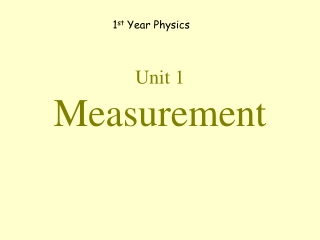DownloadDownload PresentationUnit 1 Measurement

# Unit 1 Measurement

Download Presentation## Unit 1 Measurement

- - - - - - - - - - - - - - - - - - - - - - - - - - - E N D - - - - - - - - - - - - - - - - - - - - - - - - - - -
##### Presentation Transcript

1. 1st Year Physics Unit 1Measurement

2. Instruments of Measurement • Ruler/Meter stick • Stop Clock • Thermometer • Scales • Measuring Cylinder • Opisometer • Trundle wheel • Callipers

3. Basics Units in Physics

4. Length

5. Measuring Length.

6. Using a Ruler/Metre RuleCan you spot any error being made in measuring this wire? Task: Use a ruler/meter rule to measure the length and breath of your copy in millimetres (mm)

7. Opisometer Task: Use an Opisometer & Ruler to work out the length of a curved line to the nearest cm

8. Trundle Wheel. Task: Use a Trundle Wheel to measure the length of the corridor to the nearest metre (m)

9. Vernier Calipers

10. Callipers Task: Use a callipers or a vernier calipers to measure the outside and inside diameter of a beaker in mm

11. Mass

12. Measuring Mass with a Scales Mass is the amount of matter in a substance. Unit: Kilogram (kg).

13. Temperature

14. To measure Temperatures. Thermometer Unit: Degrees Celsius (oC) Task: Use Thermometer measure the temperature of a beaker of fresh tap water.

15. Area & Volume

16. Area is the amount of surface that covers an object. 52 mm Area = Length x Breadth A = L x B 36 mm A = 52 mm x 36 mm = 1,872 mm2 Task: Measure the length and breadth of your copy and calculate its area in mm2

17. Volume is the amount of space taken up by an object. Volume = length x breadth x height. Example to calculate the volume of a matchbox V = L x B x H 36 mm 52 mm V = 36mm x 52mm x 15mm 15 mm V = 28,080 mm3 Task: Measure the dimensions of a block of wood (or matchbox) and calculate its volume in mm3

18. To measure the volume of a liquid Measuring Cylinder Liquid Volume is measured in: Litres (l) or Millilitres (ml)

19. Meniscus Read from bottom of the Meniscus

20. To measure the volume of a small object Finishing Volume Starting Volume The Increase in Volume is 25 ml So this must be the Volume of the Stone

21. To measure the Volume of an Irregular Object using an Overflow-Can Overflow Can Measuring Cylinder Task: Use an overflow-can and measuring cylinder to work out the volume of a stone in ml